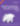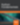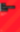# Functional Programming with Phunkie: Parser Combinators in PHP

PHP

Share:

#### Free JavaScript Book!Write powerful, clean and maintainable JavaScript.

RRP \$11.95

Phunkie is a library with functional structures for PHP. In this tutorial, Phunkie creator Marcello Duarte, head of training at Inviqa, explains how to create Parser combinators using the functional library. This post first appeared on the Inviqa blog, and was republished here with their permission.## Learning functional programming

Functional programming really matters. Pure functions (or functions with no side effect) are the perfect units of behaviour because we can rely on them to build larger and more complex systems. The greatness of functional programming relies on this power of composability.

That’s something I first came to believe back in the days of my licentiate degree on Computing for Management, during a semester in AI with Lisp. My course curriculum was mostly focused on C/C++, therefore I had to stay focused on where the skills demand was.

Thankfully, I’ve been able to reignite my love of studying functional programming here at Inviqa where we’re delivering more and more projects based on Scala, the general purpose programming language.

I’ve read “the red book” about three times (the Chiusano & Bjarnason one, not the Vaughn Vernon’s one). I took all the Martin Odersky’s Coursera courses, and I spent hours of my weekends watching videos and reading papers.

And I set myself a challenge: to do with functional programming what I did when I was learning TDD and created PHPSpec (a BDD testing and design tool for PHP developers) to help me learn; I decided to write my own functional programming dialect in PHP. And so Phunkie was born!

Now over to you. Learning functional programming means immersing yourself in a new paradigm, which can be challenging. It requires a totally different mindset for approaching problems.

So, by the time you can use functional programming to solve real-world problems, you’ll have spent hours grasping the new thinking or getting clued-up on the theory.

In this tutorial, my aim is to help fast-track your journey by going over my implementation of Hutton & Meijer’s Monadic Parser Combinators. Hopefully you will be able to see both the thinking and the usefulness of functional programming.

And, by using Phunkie, you can eliminate the need to learn Haskell to understand the topic. All you should need is some familiarity with PHP.

So let’s get started!

## Parsers

According to Terence Parr, parsing is the computer act of recognising a phrase. There are many strategies for parsing. We will be using recursive-descent parsing, one of the most basic ones, but one that’s powerful enough to implement some useful grammar constructs, such as sequencing, choice, and repetition.

## Combinators

If asked what functional programming is all about, I’d answer: composition. Functions are perfect units of behaviour that you can compose to create larger systems. Combinators are patterns of composition that have been around in functional programming long before any class or object were written. They are very elegant, too.

We will implement parsers as functions. Only three very basic parsers are enough to help us create all the other parsers in this article.

### Using types to represent functions

Let’s think of what a parser does. We give it a string. It then tries to figure out if that string matches any grammatical definition. If we have a match we keep the result as well as the remaining fragment of the string. If we fail, we stop.

So what would that function declaration look like?

``````<?php

function parse(string \$toParse): mixed
{
// if it matches a grammar, it returns the match and the remaining string
// if it doesn’t, it returns an empty set
}
``````

But a function can only return one result. So how are we returning a match plus the remaining string? We will use a Phunkie type: `Pair`. A pair allows you to group values of different types together, like so:

``````<?php

// We will use the Phunkie immutable pair for our pair
use Phunkie\Types\Pair;

function parse(string \$toParse): Pair
{
// if matches grammar
return Pair(\$match, \$remaining);
}
``````

So a parser type can be described like this: `Parser = (String) -> Pair<Result, String>`, Which reads: a parser is a function that takes a string and returns a pair composed of the parsing result and the remaining string to be parsed.Make the leap into server-side programming with a comprehensive cover of PHP & MySQL.

Normally RRP \$11.95 Yours absolutely free

This is enough if we’re only interested in one parser. But what if we’re talking about combining parsers? The result `Pair<Result, String>` is not enough, so we will have a list of those pairs, where a pair will be the result of each parser. Taking that into account, a parser type would look like: `Parser = (String) -> List<Pair<Result, String>>`.

This will be written in PHP using the class syntax in this way:

``````<?php

// We will use the Phunkie immutable list for our list
use Phunkie\Types\ImmList;

/**
* Represents a function (String) -> List<Pair<Result, String>>
*/
class Parser
{
/**
* A function that takes a String and returns List of Pairs (Result, String)
*
* @var callable
*/
private \$run;

public function __construct(callable \$run)
{
\$this->run = \$run;
}

/**
* @param string \$toParse
* @return ImmList of pairs of (results, remaining)
*/
public function run(string \$toParse): ImmList
{
return (\$this->run)(\$toParse);
}
}
``````

## Primitive parsers

Now that we have our type definition we can start creating very basic parsers. The first one is a parser that always succeeds, without consuming any of the string. Rather useless, you may say. Well, we’re just getting started, and soon enough you’ll see what we’ll be able to do with these primitive parsers.

Let’s start by creating an example of how we’d use it. We will call our parser `result`. The test would look like this:

``````<?php

describe("Parser", function() {
context("Basic parsers", function() {

describe("result", function() {

it("succeeds without consuming any of the string", function() {
expect(result("hello")->run("world"))
->toEqual(ImmList(Pair("hello", "world")));
});
});

}
}
``````

And the implementation is quite simple:

``````<?php

function result(string \$a): Parser
{
return new Parser(function(string \$s) use (\$a): ImmList {
return ImmList(Pair(\$a, \$s));
});
}
``````

That was easy. Now let’s build another primitive browser that always fails, regardless of what is given to it. A failure is represented by an empty list. An empty list in Phunkie is constructed with `Nil()`. We can call this parser: `zero`. The test would look like this:

``````        describe("zero", function(){

it("always return an empty list, which means failure", function(){
expect(zero()->run("world"))->toEqual(Nil());
});

});
``````

And you can probably guess the implementation:

``````function zero(): Parser
{
return new Parser(function(\$s): ImmList {
return Nil();
});
}
``````

Let’s cover one more basic parser. This time, a little bit more useful. This parser will consume the first character of a string. It will fail if the string is empty. Let’s see what it looks like. Here’s the example:

``````        describe("item", function() {

it("returns an empty list for an empty string", function() {
expect(item()->run(""))->toEqual(Nil());
});

it("parses a string and returns the first character", function() {
expect(item()->run("hello"))->toEqual(ImmList(Pair('h', "ello")));
});

});
``````

And the implementation should be trivial:

``````function item(): Parser
{
return new Parser(function(string \$s): ImmList {
return strlen(\$s) == 0 ? Nil() : ImmList(Pair(\$s, substr(\$s, 1)));
});
}
``````

This is it for primitive parsers. We can now capitalise on them and build combinators that combine the power of multiple parsers to build greater parsers.

## Parser combinators

Let’s imagine we want to get the string `"hello"`, apply the `item` parser, and then apply the `item` parser again. It would be nice to have a parser that lets you apply a sequence of two parsers and returns a pair of results. Let’s call that parser `seq`. Here is the example:

``````        describe("seq", function() {

it("applies parsers in sequence", function() {
expect(seq(item(), item())->run("hello"))
->toEqual(ImmList(Pair(Pair('h', 'e'), "llo")));
});

});
``````

If we try and implement this from intuition, we will probably come up with some imperative style code we are used to see outside functional programming. A first attempt would look something like this:

``````function seq(Parser \$p, Parser \$q): Parser
{
return new Parser(function(\$s) use (\$p, \$q) {
// run the first parser, store the result
\$resP = \$p->run(\$s);

// run the second parser, using the remaining string from the first parser

// return the pair with both results
});
}
``````

Looks confusing, right? That’s because it is!

This imperative style example is oversimplified. We’re not even checking if the first parser fails. What’s more, if we applied another `seq` to this, we would end up with nested tuples which is probably not a useful result for this parser.

You will remember that we defined the `Parser` type as: something which has a function that parses a string and returns a result and the remaining string. In more general terms, our `Parser` type represents a computation.

The computation can be triggered when we call the method `run`. We do this a lot in modern functional programming, using types not just to represent a family of values, but to represent other computation elements.

In fact, the operation we are after here – getting the value from within the first (parsing) context and passing that value to another (parsing) context – is a well-known pattern within functional programming.

This abstraction over a value within a context is called a monad. By implementing the monad combinator method `flatMap` on our `Parser` class we can make parsers very composable – which is the whole point of using monads.

Let me show you one possible implementation of `flatMap` for `Parser`, then we can go over it, step by step.

``````class Parser
{
private \$run;
public function __construct(callable \$run) { \$this->run = \$run; }
public function run(string \$toParse): ImmList { return (\$this->run)(\$toParse); }

public function flatMap(callable \$f): Parser
{
return new Parser(function(string \$s) use (\$f) {
return \$this->run(\$s)->flatMap(function(Pair \$result) use (\$f) {
return \$f(\$result->_1)->run(\$result->_2);
});
});
}
}
``````

What this method is doing is creating a fresh `Parser` using both its own parser function and a new parser function passed to it. First we call `run`: `\$this->run("hello")`. If `\$this` was an `item` parser, this will return something like `ImmList(Pair("h", "ello"))`.

Once we have the result of applying the Parser to the input string we can then apply the other parser function to this result. Well yes, the result is inside a list! So how do you get something from inside a context? Oh yeah, monads! Yes, lists are monads too. So we `flatMap` on the list to get access to the result: `\$this->run(\$s)->flatMap(function(Pair \$result)`. Now we can apply the given function to the result pair. We can access the first and the second element of the pair using the `_1` and `_2` read-only accessors: return `\$f(\$result->_1)->run(\$result->_2)`;.

Another important combinator is `map`. Whilst the function given to `flatMap` has to return a `Parser`, a function given to `map` can return any value, and the `map` method will make sure it is returned into the context in which the function was mapped.

``````    public function map(callable \$f)
{
return new Parser(function(string \$s) use (\$f) {
return \$this->run(\$s)->map(function(Pair \$result) use (\$f) {
return Pair(\$f(\$result->_1), \$result->_2);
});
});
}
``````

Note in the code example how the returned value of `\$f` is used to create the result of composing parser. `map` is usually used at the end of a sequence of `flatMaps`, simply to organise the values you want returned, ensuring they’re in the context you need.

Let’s consider an implementation of `seq` using monads. Note how we use `map` to expose just the result we expect to see:

``````function seq(Parser \$p, Parser \$q): Parser
{
return \$p->flatMap(function(\$x) use (\$q) {
return \$q->map(function(\$y) use (\$x) {
return Pair(\$x, \$y);
});
});
}
``````

In fact this pattern is so common in functional programming that FP languages usually have a special syntax for it. Here is how it looks in Phunkie (0.6.0):

``````function seq(Parser \$p, Parser \$q): Parser
{
return for_(
__(\$x)->_(\$p),
__(\$y)->_(\$q)
)->yields(\$x, \$y);
}
``````

I know what you’re thinking: this `seq` parser is very neat, but what’s the use of combining parsers if our parsers can only parse the first character of a string? Well, now that we’ve covered the basics using examples, we can move onto questions like this.

Stay tuned for part II in this series, which will cover more sequencing and other strategies.### Marcello Duarte

Marcello is head of training at web and software development company Inviqa, and an active contributor to open-source projects. He is the co-creator of PhpSpec, a popular BDD-flavoured testing and design tool for PHP developers, and author of Phunkie – a functional library containing most common functional structures and patterns found in Haskell (for free), based on the abstract algebra theory of categories.

#### New books out now!Learn valuable skills with a practical introduction to Python programming!Give yourself more options and write higher quality CSS with CSS Optimization Basics.